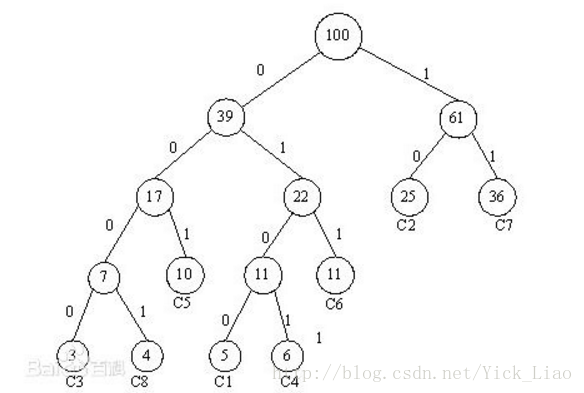# fasttext的基础理论

## 前言简介

fasttext是NLP里，一个非常高效的，基于词向量化的，用于文本分类的模型。虽然其原理比较简单，但是其中涉及到了不少的用于提速和准确率的小技巧。

## 正文

fasttext和word2vec中的CBOW非常类似，对于每一个文本而言，第一步是将所有单词向量化后作为输入；第二步是将输入的所有向量在隐藏层进行平均化处理得到新的向量；第三步输出预测值。接下来我们分别对这三部进行具体的解释。$\underset{\theta }{max}\prod _{i=1}^{d}P\left({R}_{pi}\right)$

$P\left({R}_{pi}\right)$$P(R_{pi})$指的是从${R}_{{p}_{i}}$$R_{p_i}$上一个节点（${R}_{{p}_{i-1}}$$R_{p_{i-1}}$）到它的概率，也就是上面所述的含有参数\theta的sigmoid函数。举个例子，如果${R}_{{p}_{i}}$$R_{p_i}$${R}_{{p}_{i-1}}$$R_{p_{i-1}}$的右子节点，那么$P\left({R}_{pi}\right)$$P(R_{pi})$就是$p\left(y=1\right)=\frac{1}{1+{e}^{-{\theta }_{{p}_{i-1}}w}}$$p(y=1) = \frac{1}{1+e^{-\theta _{p_{i-1}}w}}$(注意这里角标是i-1，大家想清楚是为什么，这也是为什么叶节点处不需要参数$\theta$$\theta$)

1.在中提到的对于n-grams使用hash技巧，我不是很明白是怎么个意思，原作者给了两篇参考文献还没来得及看，坐标位于中的2.2里的(Weinberger et al. 2009)和 (Mikolov et al. 2011)两篇文章。

2.对于计算方法一步中，我们更新的是向量平均值$w$$w$，但是我们最后还是希望得到每个词具体对应的向量，我们如何去得到每个词自己的词向量了？还是一开始就应该是对每个词的词向量进行更新？（因为一文中就是对这个$w$$w$进行更新，所以我就照着它写的，这个问题估计要扒源代码才能彻底明白）。

word2vector中的数学原理详解.pdf
Bag of Tricks for Efficient Text Classification（高效文本分类技巧）